# Solution June 02, 2007

### Problem

Find all polynomials of degree 3, such that for each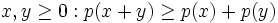$x,y\geq 0: p(x+y)\geq p(x)+p(y)$

### Solution

Let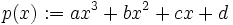$p(x):=ax^3+bx^2+cx+d\,$.

For all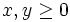$x, y\geq 0$ we have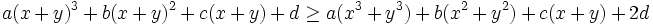$a(x+y)^3 + b(x+y)^2 +c(x+y) + d \geq a(x^3+y^3)+b(x^2+y^2)+c(x+y)+2d$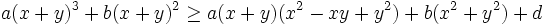$a(x+y)^3 + b(x+y)^2 \geq a(x+y)(x^2-xy+y^2)+b(x^2+y^2)+d$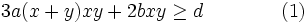$3a(x+y)xy + 2bxy \geq d \qquad \qquad (1)$

First let x = y = 0 to see that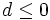$d\leq 0$.

Now set x = y and let x go to infinity we see from (1) that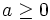$a\geq 0$.

Consider the inequality$q(t) := 6a t^3 + 2bt^2 \geq d \qquad \qquad (2)$

(2) follows from (1) for all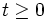$t\geq0$ by letting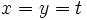$x=y=t\,$. On the other hand, if we let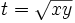$t=\sqrt{xy}$ then (1) follows from (2) and the arithmetic-geometric mean inequality,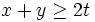$x+y\geq 2t$.

If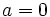$a = 0\,$, we find that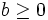$b\geq0$ by letting t go to infinity, and this suffices to satisfy (2).

Assume that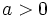$a>0\,$. We want to find a condition on d such that (2) is always satisfied. We start by minimizing q(t) for$t\geq 0$, so we set its derivative to 0: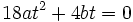$18at^2 + 4bt = 0\,$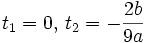$t_1 = 0,\, t_2 = -\frac{2b}{9a}$

Note that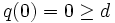$q(0)=0\geq d$ so only minima greater than 0 need to be considered.

If$b\geq0$, then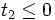$t_2\leq0$, so there are no other minima to consider.

If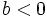$b<0\,$, then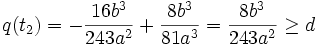$q(t_2) = -\frac{16b^3}{243a^2} + \frac{8b^3}{81a^3} = \frac{8b^3}{243a^2} \geq d$$\frac{8b^3}{243a^2}$ is negative in this case, so this is a stronger condition on d than we already had.

In summary, p(x) is a polynomial satisfying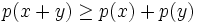$p(x+y)\geq p(x)+p(y)$ for all$x,y\geq 0$ in the following cases:

•$a\geq0$,$b\geq 0$ and$d\leq 0$
•$a > 0\,$,$b<0\,$ and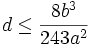$d\leq\frac{8b^3}{243a^2}$

No other possibilities for p(x) exist.